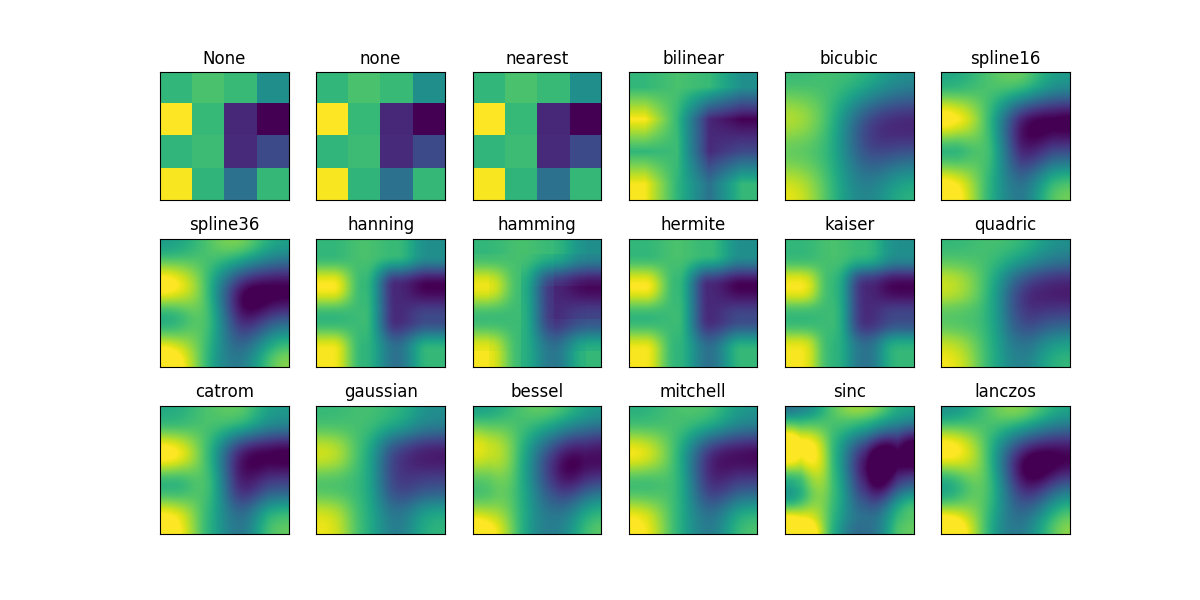Travis-CI:# Interpolations for imshow/matshow¶

This example displays the difference between interpolation methods for imshow and matshow.

If `interpolation` is None, it defaults to the rc image.interpolation parameter. If the interpolation is `none`, then no interpolation is performed for the Agg, ps and pdf backends. Other backends will default to ‘nearest’.

For the Agg, ps and pdf backends, interpolation = ‘none’ works well when a big image is scaled down, while interpolation = ‘nearest’ works well when a small image is scaled up.```import matplotlib.pyplot as plt
import numpy as np

methods = [None, 'none', 'nearest', 'bilinear', 'bicubic', 'spline16',
'spline36', 'hanning', 'hamming', 'hermite', 'kaiser', 'quadric',
'catrom', 'gaussian', 'bessel', 'mitchell', 'sinc', 'lanczos']

# Fixing random state for reproducibility
np.random.seed(19680801)

grid = np.random.rand(4, 4)

fig, axes = plt.subplots(3, 6, figsize=(12, 6),
subplot_kw={'xticks': [], 'yticks': []})